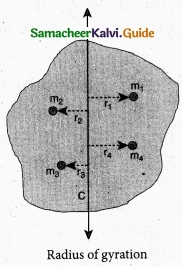Students can Download Tamil Nadu 11th Physics Model Question Paper 4 English Medium Pdf, Tamil Nadu 11th Physics Model Question Papers helps you to revise the complete Tamilnadu State Board New Syllabus, helps students complete homework assignments and to score high marks in board exams.

## TN State Board 11th Physics Model Question Paper 4 English Medium

General Instructions:

1. The question paper comprises of four parts.
2. You are to attempt all the parts. An internal choice of questions is provided wherever applicable.
3. All questions of Part I, II, III and IV are to be attempted separately.
4. Question numbers 1 to 15 in Part I are Multiple Choice Questions of one mark each.
These are to be answered by choosing the most suitable answer from the given four alternatives and writing the option code and the corresponding answer
5. Question numbers 16 to 24 in Part II are two-mark questions. These are to be answered in about one or two sentences.
6. Question numbers 25 to 33 in Part III are three-mark questions. These are to be answered in about three to five short sentences.
7. Question numbers 34 to 38 in Part IV are five-mark questions. These are to be answered in detail Draw diagrams wherever necessary.

Time: 3 Hours
Maximum Marks: 70

PART – 1

Answer all the questions: [15 × 1 = 15]

Question 1.
In some region, the gravitational field is zero. The gravitational potential in this region is …………………….
(a) A variable
(b) A constant
(c) Zero
(d) Can’t be zero
(b) A constantQuestion 2.
The angle between two vectors 2$$\hat { i }$$ + 3$$\hat { j }$$ + $$\hat { k }$$ and -3$$\hat { j }$$ + 6k is …………………..
(a) 0°
(b) 30°
(c) 60°
(d) 90°
Hint:
The angle between the two vector is 90°.
[cos θ = $$\frac{\overrightarrow{\mathrm{A}} \cdot \overrightarrow{\mathrm{B}}}{\mathrm{AB}}$$]
(d) 90°

Question 3.
A stretched rubber has
(a) Increased kinetic energy
(b) Increased potential energy
(c) Decreased kinetic energy
(d) The axis of rotation
(b) Increased potential energyQuestion 4.
The direction of the angular velocity vector is along ……………………..
(a) The tangent to the circular path
(d) The axis of rotation
(d) The axis of rotation

Question 5.
For a satellite moving in an orbit around the earth, the ratio of kinetic energy to potential energy is …………………….
(a) $$\frac{1}{2}$$
(b) $$\frac { 1 }{ \sqrt { 2 } }$$
(c) 2
(d) $$\sqrt{2}$$
Hint:(a) $$\frac{1}{2}$$Question 6.
If the linear momentum of the object is increased by 0.1 %, then the kinetic energy is increased by ………………………..
(a) 0.1%
(b) 0.2%
(c) 0.4%
(d) 0.01%
Hint:
The relation b/w linear momentum and kinetic energy
P = $$\sqrt{2 \mathrm{mE}_{\mathrm{K}}} \Rightarrow \mathrm{E}_{\mathrm{K}}=\frac{\mathrm{P}^{2}}{2 \mathrm{m}}$$
If L is increased by 0.1% 1′ = P + $$\frac{0.1}{100}$$P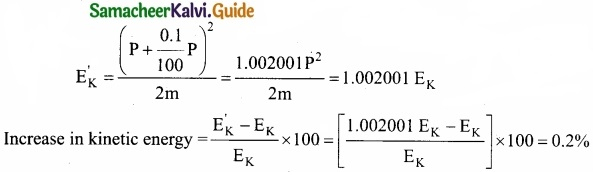(c) 0.4%

Question 7.
A block of mass 4 kg is suspended through two light spring balances A and B. Then A and B will read respectively ………………………
(a) 4 kg and 0 kg
(b) 0 kg and 4 kg
(c) 4 kg and 4 kg
(d) 2kg and 2 kg
Hint:
Tension is uniformly transmitted if the springs are massless.
(c) 4 kg and 4 kgQuestion 8.
At the same temperature, the mean kinetic energies of molecules of hydrogen and oxygen are in the ratio of ………………………
(a) 1 : 1
(b) 1 : 16
(c) 8 : 1
(d) 16 : 1
Hint:
Average kinetic energy of a molecule is proportional to the absolute temperature.
(a) 1 : 1

Question 9.
A uniform rope having mass m hangs vertically from a rigid support. A transverse wave pulse is produced at the lower end. Which of the following plots shows the correct variation of speed v with height h from the lower end?
(a)(b)(c)(d)(d)Question 10.
In a simple hormonic oscillation, the acceleration against displacement for one complete oscillation will be ………………………
(a) An ellipse
(b) A circle
(c) A parabola
(d) A straight line
(d) A straight lineQuestion 11.
A shell is fired from a canon with velocity v m/s at an angle 0 with the horizontal direction. At the highest point in the path it explodes into two pieces of equal mass. One of the pieces retraces it path of the cannon and the speed in m/s of the other piece immediately after the. explosion is ………………………..
(a) 3v cos θ
(b) 2v cos θ
(c) $$\frac{3}{2}$$v cos θ
(d) $$\frac { \sqrt { 3 } }{ 2 }$$ cos θ
Hint:
Velocity at the highest point = horizontal component of velocity = V cos θ
Momentum of shell before explosion = mv cos θ
Momentum of two pieces after explosion = $$\frac{m}{2}$$ (- V cos θ) + $$\frac{m}{2}$$ v
Law of conservation of momentum mv cos θ = –$$\frac{mv}{2}$$cos θ + $$\frac{m}{2}$$ v
∴ V = 3V cos θ
(a) 3v cos θ

Question 12.
In which process, the p – v indicator diagram is a straight line parallel to volume axis?
(a) Isothermal
(c) Isobaric
(d) Irreversible
(c) IsobaricQuestion 13.
For a liquid to rise in capillary tube, the angle of contact should be ………………………….
(a) Acute
(b) Obtuse
(c) Right
(d) None of these
(a) Acute

Question 14.
The increase in internal energy of a system is equal to the work done on the system which process does the system undergo?
(a) Isochoric
(c) Isobaric
(d) IsothermalQuestion 15.
The change in frequency due to Doppler effect does not depend on …………………………
(a) The speed of the source
(b) The speed of the observer
(c) The frequency of the source
(d) Separation between the source and the observer
(d) Separation between the source and the observer

PART – II

Answer any six questions in which Q. No 23 is compulsory. [6 × 2 = 12]

Question 16.
What are the advantages of SI system?

1. This system makes use of only one unit for one physical quantity, which means a rational system of units
2. In this system, all the derived units can be easily obtained from basic and supplementary units, which means it is a coherent system of units.
3. It is a metric system which means that multiples and submultiples can be expressed as powers of 10.Question 17.
Using the principle of homogunity of dimensions, check dimensionally the given equations are correct,
(a) $$\mathbf{T}^{2}=\frac{4 \pi^{2} r^{3}}{\mathbf{G}}$$
(b) $$T^{2}=\frac{4 \pi^{2} r^{3}}{G M}$$?
Here G-gravitational constant
r – radius of orbit M – mass
Dimensional formula for T = T
Dimensional formula for r = L
Dimensional formula for G = M-1L3T-2
Dimensional formula for M = M
(a) T2 M° L° = [L3] [M L-3T-2]
T2 M° L° = L° MT2 – Not correct

(b) T2 = [L3][ML-3T2][M-1]
T2 = T2 – Dimensionally correct

Question 18.
Find out the workdone required to extract water from the well of depth 20 m. Weight of water and backet is 2.8 kg wt?
Workdone, W = mgh
W = (Weight) × depth
= 2.8 × 20
W = 56 JQuestion 19.
Is a single isolated force possible in nature?
No. According to Newton’s third law, for every action, there is an equal and opposite reaction. So, whatever case we consider, if there is an action there is always a reaction. So it is impossible.

Question 20.
State the factors on which the moment of inertia of a body depends?

1. Mass of body
2. Size and shape of body
3. Mass distribution w.r.t. axis of rotation
4. Position and orientation of rotational axis

Question 21.
If a drop of water falls on a very hot iron, it takes long time to evaporate. Explain why?
When a drop of water falls on a very hot iron it gets insulated from the hot iron due to a thin layer of water vapour which is a bad conductor of heat. It takes quite long to evaporate as heat is conducted from hot iron to the drop through the insulating layer of water vapour very slowly.Question 22.
What is meant by gravitational field. Give its unit?
The gravitational field intensity $$\vec { E }$$1, at a point is defined as the gravitational force experienced by unit mass at that point. It’s unit N kg-1.

Question 23.
Why dimensional methods are applicable only up to three quantities?
Understanding dimensions is of utmost importance as it helps us in studying the nature of physical quantities mathematically. The basic concept of dimensions is that we can add or subtract only those quantities which have same dimensions. Also, two physical quantities are equal if they have same dimensions, these basic ideas help us in deriving the new relation between physical quantities, it is just like units.Question 24.
Why does a porter bend forward while carrying a sack of rice on his back?
When a porter carries a sack of rice, the line of action of his centre of gravity will go away from the body. It affects the balance, to avoid this he bends. By which centre of gravity will realign within the body again. So balance is maintained.

PART – III

Answer any six questions in which Q.No. 29 is compulsory. [6 × 3 = 18]

Question 25.
Explain variation of ‘g’ with latitude?
When an object is on the surface for the Earth, it experiences a centrifugal force that depends on the latitude of the object on Earth. If the Earth were not spinning, the force on the object would have been mg. However, the object experiences an additional centrifugal force due to spinning of the Earth.
OPz, cos λ = $$\frac{PZ}{OP}$$ = $$\frac{R’}{R}$$
R’ = R cos λwhere λ is is the latitude. The component of centrifugal acceleration experienced by the object in the direction opposite to g is
aPQ = ω2R cos λ = ω2R cos2 λ
Since R’ = R cos λ
Therefore, g’ = g – ω’2R cos2λ
From the above expression, we can infer that at equator, λ = 0, g’ = g – ω2R. The acceleration due to gravity is minimum. At poles λ = 90; g1 = g. it is maximum. At the equator, g’ is minimum.Question 26.
Cap you associate a vector with
(a) the length of a wire bent into a loop
(b) a plane area
(c) a sphere.
(a) We cannot associate a vector with the length of a wire bent into a loop, this is cause the length of the loop does not have a definite direction.

(b) We can associate a vector with a plane area. Such a vector is called area vector and its direction is represented by a normal drawn outward to the area.

(c) The area of a sphere does not point in any difinite direction. However, we can associate a null vector with the area of the sphere. We cannot associate a vector with the volume of a sphere.Question 27.
What is mean by inertia? Explain its types with example?
The inability of objects to move on its own or change its state of motion is called inertia.
Inertia means resistance to change its state. There are three types of inertia:

1. Inertia of rest: The inability of an object to change its state of rest is called inertia of rest.
Example:

• When a stationary bus starts to move, the passengers experience a sudden backward push.
• A book lying on the table will remain at rest until it is moved by some external agencies.

2. Inertia of motion: The inability of an object to change its state of uniform speed (constant speed) on its own is called inertia of motion.
Example:

• When the bus is in motion, and if the brake is applied suddenly, passengers move forward and hit against the front seat.
• An athlete running in a race will continue to run even after reaching the finishing point.

3. Inertia of direction: The inability of an object to change its direction of motion on its own is called inertia of direction.
Example:

• When a stone attached to a string is in whirling motion, and if the string is cut suddenly, the stone will not continue to move in circular motion but moves tangential to the circle.
• When a bus moving along a straight line takes a turn to the right. The passengers are thrown towards left.Question 28.
A light body and body with greater mass both are having equal kinetic energy. Among these two which one will have greater linear momentum?
Given Data:
E1 – E2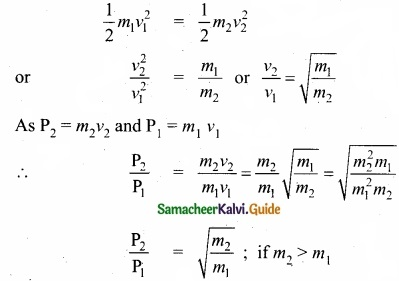then P2 > P1
i.e; a heavier body has greater linear momemtum.

Question 29.
Three particles of masses m1 = 1 kg, m2 = 2 kg and m3 = 3 kg are placed at the corners of an equilateral triangle of side lm as shown in Figure. Find the position of center of mass?
The center of mass of an equilateral triangle lies at its geometrical center G.
The positions of the mass m1, m2 and m3 are at positions A, B and C as shown in the Figure. From the given position of the masses, the coordinates of the masses m1 and m2 are easily marked as (0,0) and (1,0) respectively.

To find the position of m3 the Pythagoras theorem is applied. As the ∆DBC is a right angle triangle,
BC2 = CD2 + DB2
CD2 = BC2 – DB2
CD2 = 12 – ($$\frac{1}{2}$$)2 = 1 – ($$\frac{1}{4}$$) = $$\frac{3}{4}$$
CD = $$\frac { \sqrt { 3 } }{ 2 }$$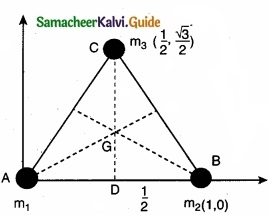The position of mass m3 is or (0.5, 0.5$$\sqrt{3}$$)
X coordìnate of center of mass,
yCM = $$\frac{m_{1} y_{1}+m_{2} y_{2}+m_{3} y_{3}}{m_{1}+m_{2}+m_{3}}$$
yCM = $$\frac { \sqrt { 3 } }{ 4 }$$ m
∴ The coordinates of center of mass G (xCM, yCM) is ($$\frac{7}{12}$$, $$\frac { \sqrt { 3 } }{ 4 }$$)Question 30.
Define precision and accuracy. Explain with one example?
The accuracy of a measurement is a measure of how close the measured value is to the true value of the quantity. Precision of a measurement is a closeness of two or more measured values to each other.

The true value of a certain length is near 5.678 cm. In one experiment, using a measuring instrument of resolution 0.1 cm, the measured value is found to be 5.5 cm. In another experiment using a measuring instrument of greater resolution, say 0.01 cm, the length is found to be 5.38 cm. We find that the first measurement is more accurate as it is closer to the true value, but it has lesser precision. On the contrary, the second measurement is less accurate, but it is more precise.

Question 31.
Derive an expression for total acceleration in the non uniform circular motion?
If the speed of the object in circular motion is not constant, then we have non-uniform circular motion. For example, when the bob attached to a string moves in vertical circle, the speed of the bob is not the same at all time. Whenever the speed is not same in circular motion, the particle will have both centripetal and tangential acceleration as shown in the figure.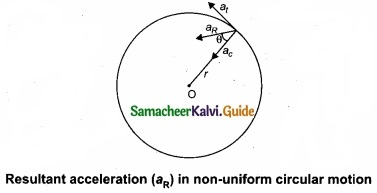The resultant acceleration is obtained by vector sum of centripetal and tangential acceleration. Since centripetal acceleration is $$\frac { v^{ 2 } }{ r }$$, the magnitude of this resultant acceleration is given by
aR = $$\sqrt{a_{t}^{2}+\left(\frac{v^{2}}{r}\right)^{2}}$$

This resultant acceleration makes an angle θ with the radius vector as shown in figure.
This angle is given by tan θ = $$\frac{a_{t}}{\left(v^{2} / r\right)}$$Question 32.
Calculate the value of adiabatic exponent for monoatomic molecule?
Monoatomic molecule:
Average kinetic energy of a molecule = [$$\frac{3}{2}$$kT]
Total energy of a mole of gas = $$\frac{3}{2}$$ kT × NA = $$\frac{3}{2}$$RT
For one mole, the molar specific heat at constant volumeQuestion 33.
At what temperature will the rms speed of oxygen molecules become just sufficient for escaping from the Earth’s surface, (mass of oxygen molecule : 2.76 × 10-26kg Boltzmann’s constant (kB) = 1.38 × 10-23 J mol-1 k-1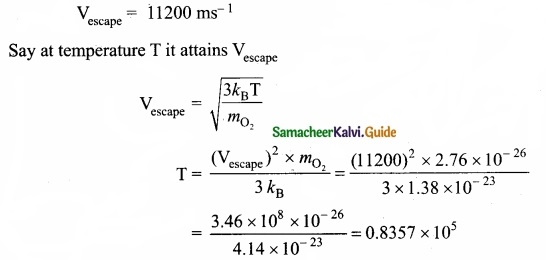PART – IV

Answer all the questions. [5 × 5 = 25]

Question 34 (a).
State kegler’s laws of planetary motion?
1. Law of Orbits:
Each planet moves around the Sun in an elliptical orbit with the Sun at one of the foci.

2. Law of area:
The radial vector (line joining the Sun to a planet) sweeps equal areas in equal intervals of time.

3. Law of period:
The square of the time period of revolution of a planet around the Sun in its elliptical orbit is directly proportional to the cube of the semi-major axis of the ellipse.
T2 ∝ a3
$$\frac { T^{ 2 } }{ a^{ 3 } }$$ = Constant

(b) The distance of planet Jupiter from the sun is 5.2 times that of the earth. Find the period of resolution of Jupiter around the sun?
Here r1 = 5.2 re; TJ = ?; Te = 1 year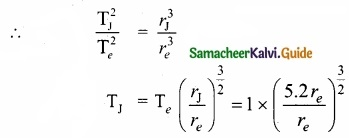= 11.86 years

[OR]

(c) Explain the propagation of errors in multiplication?
Error in the product of two quantities:
Let ∆A and ∆B be the absolute errors in the two quantities A, and B, respectively. Consider the product Z = AB,
The error AZ in Z is given by Z ± AZ = (A ± ∆A) (B ± ∆B)
= (AB) ± (A ∆ B) ± (B ∆ A) ± (∆A • ∆B)
Dividing L.H.S by Z and R.H.S by AB, we get,
1 ± $$\frac{∆Z}{Z}$$ = 1 ± $$\frac{∆B}{B}$$ ± $$\frac{∆A}{A}$$ ± $$\frac{∆A}{A}$$. $$\frac{∆B}{B}$$
As ∆A/A, ∆B/B are both small quantities, their product term $$\frac{∆A}{A}$$.$$\frac{∆B}{B}$$ can be neglected.
The maximum fractional error in Z is
$$\frac{∆Z}{Z}$$ = ± ($$\frac{∆A}{A}$$ + $$\frac{∆B}{B}$$)

(d) The period of oscillation of a simple pendulum in the experiment is recorded as 2.63 s, 2.56 s, 2.42s, 271s and 2.80s respectively. Calculate the average absolute error?
Mean absolute error = $$\frac{\Sigma\left|\Delta \mathrm{T}_{i}\right|}{n}$$
∆Tm = $$\frac{0.01+0.06+0.20+0.09+0.18}{5}$$
∆Tin = $$\frac{0.54}{5}$$ = 0.108s = 0.1 1s (Rounded of 2nd decimal place).Question 35 (a).
Explain in detail the triangle law of vector addition?
Let us consider two vectors $$\vec { A }$$ and $$\vec { B }$$ as shown in figure.

To find the resultant of the two vectors we apply the triangular law of addition as follows:

Represent the vectors $$\vec { A }$$ and $$\vec { B }$$ by the two adjacent sides of a triangle taken in the same order. Then the resultant is given by the third side of the triangle as shown in figure.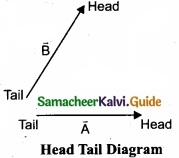To explain further, the head of the first vector $$\vec { A }$$ is connected to the tail of the second vector $$\vec { B }$$. Let θ be the angle between $$\vec { A }$$ and $$\vec { B }$$. Then $$\vec { R }$$ is the resultant vector connecting the tail of the first vector A to the head of the second vector B.

The magnitude of R (resultant) is given geometrically by the length of $$\vec { R }$$ (OQ) and the direction of the resultant vector is the angle between $$\vec { R }$$ and $$\vec { A }$$. Thus we write $$\vec { R }$$ = $$\vec { A }$$ + $$\vec { B }$$.

1. Magnitude of resultant vector:
The magnitude and angle of the resultant vector are determined by using triangle law of vectors as follows. From figure, consider the triangle ABN, which is obtained by extending the side OA to ON. ABN is a right angled triangle.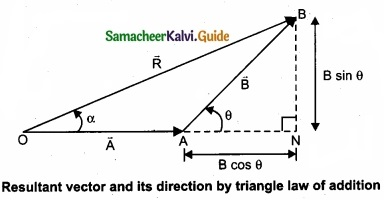From figure, let R is the magnitude of the resultant of $$\vec { A }$$ and $$\vec { B }$$.
cos θ = $$\frac{AN}{B}$$ ∴AN = B cos θ ans sin θ = $$\frac{BN}{B}$$ ∴ BN = B sin θ
For ∆OBN, we have OB2 = ON2 + BN2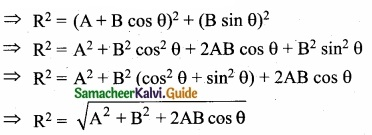2. Direction of resultant vectors: If θ is the angle between $$\vec { A }$$ and $$\vec { B }$$, then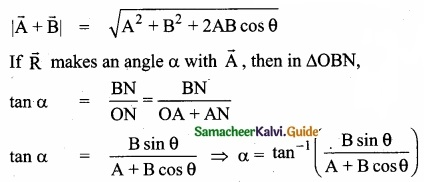(b) State and prove Bernoulli’s theorem for a flow of incompressible, non-viscous, and streamlined flow of fluid?
Bernoulli’s theorem:
According to Bernoulli’s theorem, the sum of pressure energy, kinetic energy, and potential energy per unit mass of an incompressible, non-viscous fluid in a streamlined flow remains a constant. Mathematically,
$$\frac { P }{ \rho }$$ + $$\frac{1}{2}$$v2 + gh = Constant
This is known as Bernoulli’s equation.

Proof: Let us consider a flow of liquid through a pipe AB. Let V be the volume of the liquid when it enters A in a time t which is equal to the volume of the liquid leaving B in the same time. Let aA, vA and PA be the area of cross section of the tube, velocity of the liquid and pressure exerted by the liquid at A respectively.

Let the force exerted by the liquid at A is
FA = PAaA

Distance travelled by the liquid in time t is d = vat

Therefore, the work done is W = FAd = PAaAvAt
But aAvAt = aAd = V, volume of the liquid entering at A.

Thus, the work done is the pressure energy (at A), W = PAd = PAV
Pressure energy per unit volume at
A = $$\frac{\text { Pressure energy }}{\text { Volume }}$$ = $$\frac{P_{A} V}{V}$$ = PA

Pressure energy per unit mass
A = $$\frac{\text { Pressure energy }}{\text { Mass }}$$ = $$\frac{P_{A} V}{m}$$ = $$\frac{P_{A}}{\frac{m}{V}}=\frac{P_{A}}{\rho}$$

Since m is the mass of the liquid entering at A in a given time, therefore, pressure energy of the liquid at A is
EPA = PAV = PAV × ($$\frac{m}{m}$$) = m$$\frac { P_{ A } }{ \rho }$$

Potential energy of the liquid at A,
PEA = mg hA

Due to the flow of liquid, the kinetic energy of the liquid at A,
KEA = $$\frac{1}{2} m v_{\mathrm{A}^{2}}$$

Therefore, the total energy due to the flow of liquid at A,
EA = EPA + KEA + PEA
EA = m $$m \frac{P_{A}}{\rho}+\frac{1}{2} m v_{A}^{2}+m g h_{A}$$

Similarly, let aB, vB and PB be the area of cross section of the tube, velocity of the liquid and pressure exerted by the liquid at B. Calculating the total energy at FB, we get
EB = m $$m \frac{P_{B}}{\rho}+\frac{1}{2} m v_{B}^{2}+m g h_{B}$$
From the law of conservation of energy,
EA = EB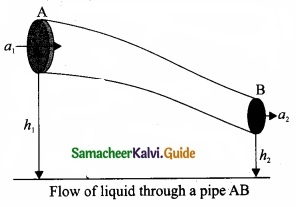Thus, the above equation can be written as
$$\frac{P}{\rho g}+\frac{1}{2} \frac{v^{2}}{g}$$ + h = Constant

The above equation is the consequence of the conservation of energy which is true until there is no loss of energy due to friction. But in practice, some energy is lost due to friction.

This arises due to the fact that in a fluid flow, the layers flowing with different velocities exert frictional forces on each other. This loss of energy is generally converted into heat energy. Therefore, Bernoulli’s relation is strictly valid for fluids with zero viscosity or non-viscous liquids. Notice that when the liquid flows through a horizontal pipe, then
h = 0 ⇒ $$\frac{P}{\rho g}+\frac{1}{2} \frac{v^{2}}{g}$$ = ConstantQuestion 36 (a).
What are the limitations of dimensional analysis?
Limitations of Dimensional analysis

1. This method gives no information about the dimensionless constants in the formula like 1, 2, …………….. π, e, etc.
2. This method cannot decide whether the given quantity is a vector or a scalar.
3. This method is not suitable to derive relations involving trigonometric, exponential and logarithmic functions.
4. It cannot be applied to an equation involving more than three physical quantities.
5. It can only check on whether a physical relation is dimensionally correct but not the correctness of the relation.

For example, using dimensional analysis, s = ut + $$\frac{1}{3}$$at2 is dimensionally correct whereas the correct relation is s = ut + $$\frac{1}{2}$$at2

(b) The escape velocity v of a body depends on

1. The acceleration due to gravity ‘g’ of the planet
2. The radius R of the planet. Establish dimensionally the relation for the escape velocity?

$$v \propto g^{a} \mathrm{R}^{b} \Rightarrow v=k g^{a} \mathrm{R}^{b}$$, K → dimensionally proportionality constant.
[v] = [g]a [R]b
[M0L1T-1] = [M0L1T-2]a [M0L1T10]b
equating powers
1 = a + b
-1 = -2a ⇒ a = $$\frac{1}{2}$$
b = 1 – a = 1 – $$\frac{1}{2}$$ = $$\frac{1}{2}$$
∴ v = k$$\sqrt{gR}$$

[OR]

(c) Discuss the law of transverse vibrations In stretched string?
Laws of transverse vibrations in stretched strings:
There are three laws of transverse vibrations of stretched strings which are given as follows:

(I) The law of length:
For a given wire with tension T (which is fixed) and mass per unit length p (fixed) the frequency varies inversely with the vibrating length. Therefore,
f ∝$$\frac{1}{l}$$ ⇒ f = $$\frac{C}{2}$$
⇒1 × f = C, where C is constant

(II) The law of tension:
For a given vibrating length I (fixed) and mass per unit length p (fixed) the frequency varies directly with the square root of the tension T,
f ∝$$\sqrt{T}$$
⇒f = A$$\sqrt{T}$$, where A is constant

(III) The law of mass:
For a given vibrating length l (fixed) and tension T (fixed) the frequency varies inverely with the square root of the mass per unit length µ,
f = $$\frac{1}{\sqrt{\mu}}$$
⇒f = $$\frac{B}{\sqrt{\mu}}$$, where B is constant

(d) Explain how to determine the frequency of tuning for k using sonometer?
Working: A transverse stationary or standing wave is produced and hence, at the knife edges P and Q, nodes are formed. In between the knife edges, anti-nodes are formed. If the length of the vibrating element is l then
l = $$\frac{\lambda}{2}$$ ⇒ λ = 2l
Let f be the frequency of the vibrating element, T the tension of in the string and µ the mass per unit length of the string. Then using equation ,we get
f = $$\frac{v}{\lambda}=\frac{1}{2 l} \sqrt{\frac{T}{\mu}}$$ in Hertz …………………. (1)

Let ρ be the density of the material of the string and d be the diameter of the string. Then the mass per unit length µ,Question 37.
(a) To move an object, which one is easier, push or pull? Explain?
When a body is pushed at an arbitrary angle θ (o to $$\frac{π}{2}$$), the applied force F can be resolved into two components as F sin e parallel to the surface and F cos θ perpendicular to the surface as shown in figure. The total downward force acting on the body is mg + F cos θ. It implies that the normal force acting on the body increases. Since there is
no acceleration along the vertical direction the normal force N is equal to
Npush = mg + F cos θ ………………………. (1)
As a result the maximal static friction also increases and is equal to
$$f_{s}^{\max }=\mu_{r} N_{p u s h}=\mu_{s}(m g+F \cos \theta)$$ ………………… (2)
Equation (2) shows that a greater force needs to be applied to push the object into motion.
When an object is pulled at an angle θ, the applied force is resolved into two components as shown in figure. The total downward force acting on the object is
Npull = mg – F cos θ ……………….. (3)Equation (3) shows that the normal force needs is less than Npush. From equations (1) and (3), it is easier to pull an object than to push to make it move.

[OR]

(b) Derive an expression for the velocities of two objects colliding elastically in one dimension?
Consider two elastic bodies of masses m1 and m2 moving in a straight line (along positive x direction) on a frictionless horizontal surface as shown in figure.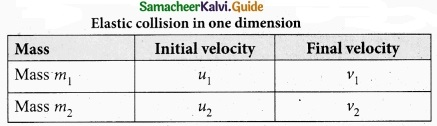In order to have collision, we assume that the mass m1 moves, faster than mass m2 i.e., u1 > u2. For elastic collision, the total linear momentum and kinetic energies of the two bodies before and after collision must remain the same.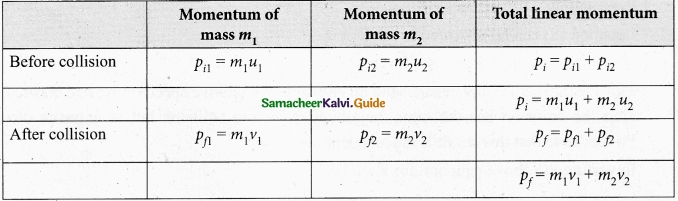From the law of conservation of linear momentum,
Total momentum before collision (ρi) = Total momentum after collision (ρf)
m1u1 + m2u2 = m1v1 + m2 v1 …………………. (1)
or m1 (u1 – v1) = m2(v2 – u2) …………………… (2)
Furthur,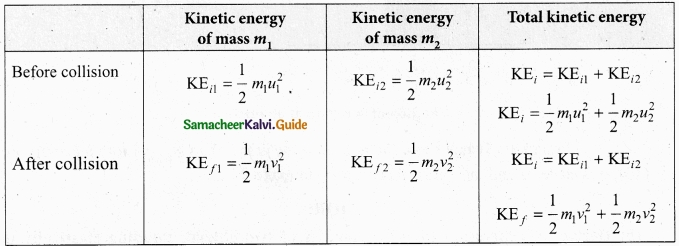For elastic collision,
Total kinetic energy before collision KEi = Total kinetic energy after collision KEf
$$\frac{1}{2} m_{1} u_{1}^{2}+\frac{1}{2} m_{2} u_{2}^{2}=\frac{1}{2} m_{1} v_{1}^{2}+\frac{1}{2} m_{2} v_{2}^{2}$$ ………………….. (3)
$$m_{1}\left(u_{1}^{2}-v_{1}^{2}\right)=m_{2}\left(v_{2}^{2}-u_{2}^{2}\right)$$
After simplifying and rearranging the terms,
Using the formula a2 – b2 = (a + b) (a – b), we can rewrite the above equation as
$$m_{1}\left(u_{1}+v_{1}\right)\left(u_{1}-v_{1}\right)=m_{2}\left(v_{2}+u_{2}\right)\left(v_{2}-u_{2}\right)$$ ……………….. (4)
Dividing the equation (4) by (2) we get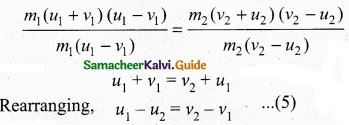Equation (5) can be written as
u1 – u2 = -(v1 – v2)
This means that for any elastic head on collision, the relative speed of the two elastic bodies after the collision has the same magnitude as before collision but in opposite direction. Further note that this result is independent of mass.
Rewriting the above equation for v1 and v2,
v1 = v2 + u2 – u1 …………………….. (6)
or v2 = u1 + v1 – u1 …………………….. (7)
To find the final velocities V1 and v2:
Substituting equation (5) in equation (2) gives the velocity of m1 as
m1 (u1 – v1) = m2 (u1 + v1 – u2 – u2)
m (u1 – v1) = m2 (u1 + v1 – 2u2)
m1u1 – m1v1 = m2u1 + m2v1 + 2m2u2
m1u1 – m2u1 + 2m2u2 = m1v1 + m2v1
(m1 – m2)u1 + 2m2u2 = (m1 + m2) v1
or v1 = $$\left(\frac{m_{1}-m_{2}}{m_{1}+m_{2}}\right)$$ u1  + $$\left(\frac{2 m_{2}}{m_{1}+m_{2}}\right)$$ u2
Similarly, by substituting (6) in equation (2) or substituting equation (8) in equation (7), we get the final m2 as
v2 = $$\left(\frac{2 m_{1}}{m_{1}+m_{2}}\right)$$ u1 + $$\left(\frac{m_{2}-m_{1}}{m_{1}+m_{2}}\right)$$ u2 …………………. (9)Question 38 (a).
Prove that at points near the surface of the Earth the gravitational potential energy of the object is v = mgh?
When an object of mass m is raised to a height h, the potential energy stored in the object is mgh. This can be derived using the general expression for gravitational potential energy. Consider the Earth and mass system, with r, the distance between the mass m and the Earth’s centre. Then the gravitational potential energy.
U = –$$\frac{\mathrm{GM}_{e} m}{r}$$ …………………… (1)Here r = Re + h, where Re is the radius of the Earth, h is the height above the Earth’s surface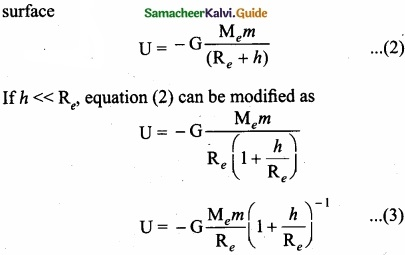By using Binomial expansion and neglecting the higher order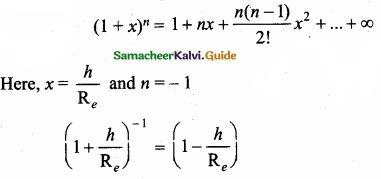Replace this value and we get,
U = $$-\frac{\mathrm{GM}_{e} m}{\mathrm{R}_{e}}\left(1-\frac{h}{\mathrm{R}_{e}}\right)$$ ………………… (4)

We know that, for a mass m on the Earth’s surface,
$$\mathrm{G} \frac{\mathrm{M}_{e} m}{\mathrm{R}_{e}}=m g \mathrm{R}_{e}$$ …………………… (5)

Substituting equation (4) in (5) we get,
U = -mgRe + mgh ………………….. (6)

It is clear that the first term in the above expression is independent of the height h. For example, if the object is taken from height h1 to h2, then the potential energy at h1 is
U(h1) = -mgRe + mgh1 …………………… (7)
and the potential energy at h2 is
U(h2) = -mgRe + mgh2 …………………… (8)

The potential energy differenqe between h1 and h2 is
U(h2) – U(h1) = mg(h1-h2) …………………. (9)

The term mgRe in equation (7) and (8) plays no role in the result. Hence in the equation (6) the first term can be omitted or taken to zero. Thus it can be stated that the gravitational potential energy stored in the particle of mass m at a height h from the surface of the Earth is U = mgh. On the surface of the Earth, U = 0, since h is zero.

[OR]

(b) Derive an expression for Radius of gyration?
For bulk objects of regular shape with uniform mass distribution, the expression for moment of inertia about an axis involves their total mass and geometrical features like radius, length, breadth, which take care of the shape and the size of the objects.

But, we need an expression for the moment of inertia which could take care of not only the mass, shape and size of objects, but also its orientation to the axis of rotation. Such an expression should be general so that it is applicable even for objects of irregular shape and non-uniform distribution of mass. The general expression for moment of inertia is given as,
I = MK2

where, M is the total mass of the object and K is called the radius of gyration. The radius of gyration of an object is the perpendicular distance from the axis of rotation to an equivalent point mass, which would have the same mass as well as the same moment of inertia of the object.

As the radius of gyration is distance, its unit is m. Its dimension is L. A rotating rigid body with respect to any axis, is considered to be made up of point masses m1, m2, m3, . . . mn at perpendicular distances (or positions) r1, r2, r3 . . . rn respectively as shown in figure. The moment of inertia of that object can be written as,

I = $$\sum m_{1} r_{1}^{2}=m_{1} r_{1}^{2}+m_{2} r_{2}^{2}+m_{3} r_{3}^{2}+\ldots .+m_{n} r_{n}^{2}$$
If we take all the n number of individual masses to be equal
m = m1 = m, = m2 = m3 = ……………… = mn
thenI = MK2

where, nm is the total mass M of the body and K is the radius of gyration.

The expression for radius of gyration indicates that it is the root mean square (rms) distance of the particles of the body from the axis of rotation. In fact, the moment of inertia of any object could be expressed in the form, I = MK2

For example, let us take the moment of inertia of a uniform rod of mass M and length l. Its moment of inertia with respect to a perpendicular axis passing through the center of mass is,
I = $$\frac{1}{12}$$Ml2
In terms of radius of gyration, I = MK2
Hence, MK2 = $$\frac{1}{12}$$Ml2
K2 = $$\frac{1}{12}$$l2
K = $$\frac{1}{12}$$ or K = $$\frac{1}{2 \sqrt{3}} l$$ or K = (0.289)l